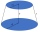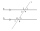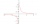# Rectangle

The rectangle is 21 cm long and 38 cm wide.

Determine the radius of the circle circumscribing rectangle.

Result

r =  22 cm

#### Solution:

$a = 21 \ cm \ \\ b = 38 \ cm \ \\ \ \\ u = \sqrt{ a^2+b^2 } = \sqrt{ 21^2+38^2 } = \sqrt{ 1885 } \ cm \doteq 43.4166 \ cm \ \\ \ \\ D = u = 43.4166 = \sqrt{ 1885 } \ cm \doteq 43.4166 \ cm \ \\ \ \\ r = D/2 = 43.4166/2 \doteq 21.7083 = 22 \ \text { cm }$

Leave us a comment of this math problem and its solution (i.e. if it is still somewhat unclear...):

Showing 1 comment:Pancake
Thanks, this really helped. Ima go eat my French toast now#### Following knowledge from mathematics are needed to solve this word math problem:

Do you want to convert length units? Pythagorean theorem is the base for the right triangle calculator. See also our trigonometric triangle calculator.

## Next similar math problems:

1. A rectangle 2A rectangle has a diagonal length of 74cm. It’s sides are 5:3. Find its lengths.
2. Circular railwayThe railway is to interconnect in a circular arc the points A, B, and C, whose distances are | AB | = 30 km, AC = 95 km, BC | = 70 km. How long will the track from A to C?
3. Secret treasureScouts have a tent in the shape of a regular quadrilateral pyramid with a side of the base 4 m and a height of 3 m. Determine the radius r (and height h) of the container so that they can hide the largest possible treasure.
4. A rhombusA rhombus has sides of length 10 cm, and the angle between two adjacent sides is 76 degrees. Find the length of the longer diagonal of the rhombus.
5. Frustum of a coneA reservoir contains 28.54 m3 of water when completely full. The diameter of the upper base is 3.5 m while at the lower base is 2.5 m. Determine the height if the reservoir is in the form of a frustum of a right circular cone.
6. Heptagonal pyramidA hardwood for a column is in the form of a frustum of a regular heptagonal pyramid. The lower base edge is 18 cm and the upper base of 14 cm. The altitude is 30 cm. Determine the weight in kg if the density of the wood is 0.10 grams/cm3.
7. Before yesterdayHe merchant adds a sale sign in his shop window to the showed pair of shoes in the morning: "Today by p% cheaper than yesterday. " After a while, however, he decided that the sign saying: "Today 62.5% cheaper than the day before yesterday". Determine the n
8. Isosceles triangle 10In an isosceles triangle, the equal sides are 2/3 of the length of the base. Determine the measure of the base angles.
9. The hemisphereThe hemisphere container is filled with water. What is the radius of the container when 10 liters of water pour from it when tilted 30 degrees?
10. Parallels and one secantThere are two different parallel lines a, b and a line c that intersect the two parallel lines. Draw a circle that touches all lines at the same time.
11. Cylinder and its circumferenceIf the height of a cylinder is 4 times its circumference. What is the volume of the cylinder in terms of its circumference, c?
12. Sides of right angled triangleOne leg is 1 m shorter than the hypotenuse, and the second leg is 2 m shorter than the hypotenuse. Find the lengths of all sides of the right-angled triangle.
13. Coordinates of square verticesI have coordinates of square vertices A / -3; 1/and B/1; 4 /. Find coordinates of vertices C and D, C 'and D'. Thanks Peter.
14. Square sideIf we enlarge the square side a = 5m, its area will increase by 10,25%. How many percent will the side of the square increase? How many percent will it increase the circumference of the square?
15. Surface of the cylinderCalculate the surface of the cylinder for which the shell area is Spl = 20 cm2 and the height v = 3.5 cm
16. Compound interest 3After 8 years, what is the total amount of a compound interest investment of \$25,000 at 3% interest, compounded quarterly? (interest is now dream - in the year 2019)
17. Equation 23Find value of unknown x in equation: x+3/x+1=5 (problem finding x)